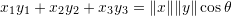### Linear Vector Spaces: Additional Structure for Linear Vector Spaces

#### Learning Outcomes

• Calculate the norm of vectors, the dot product, and the distance between vectors
• Identify the relationship between the Mathematical definition and the associated geometry of linear vector spaces

### Norm

Vector spaces can be endowed with a function called the “Norm” function. The norm function is used to describe the size of vectors in a linear vector space. It assigns strictly positive values for nonzero vectors.

Let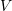be a linear vector space over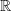. A norm is defined as a function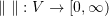that satisfies the following properties.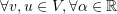:

1. Positive homogeneity or positive scalability: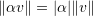2. Triangle inequality: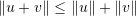3. Positive definiteness: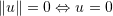There are many candidate functions that satisfy the above definition. The “Euclidean Norm” is by far the most commonly used norm function. The Euclidean norm is defined as: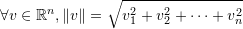##### Exercises:
• Show that the triangle inequality is equivalent to: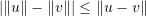• Show that the Euclidean norm satisfies the properties of a norm function
• Show that the function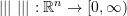such that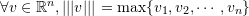satisfies the properties of a norm function

### Metric (Distance)

Vector spaces can be endowed with a “Metric” function. The metric function is used to define a distance between any two vectors in a linear vector space. Letbe a linear vector space over. A metric is defined as a function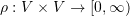that satisfies the following properties.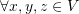:

1. Symmetry: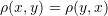2. Triangle inequality: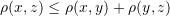3.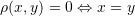There are many candidate functions that satisfy the above definition. Usually, the metric function can be induced from the norm function by setting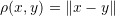. The “Euclidean Metric” is a commonly used metric function and is generated by the “Euclidean Norm”:##### Exercises:
• Show that the Euclidean metric satisfies the properties of a metric function.
• Show that the functiondefined as:satisfies the properties of a metric function.

### Inner Product

Vector spaces can be endowed with a function called the Inner Product function which associates each pair of vectors with a scalar quantity. The inner product function allows adding geometric structure to the vector space by defining orthogonality and angles. Letbe an linear vector spaces over the field of complex numbers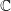. The inner product is a map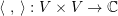such that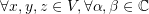:

1. Conjugate symmetry: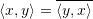2. Linearity in the first argument: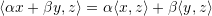3. Positive definiteness: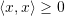with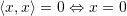Whenis defined over the field of real numbers, the inner product becomes a bilinear map and conjugate symmetry becomes symmetry.

#### Euclidian Dot Product

The “Euclidean Dot Product” is one example of an inner product function. The dot product is defined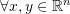as follows: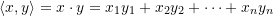The “Euclidean Dot Product” is related to the “Euclidean Norm” as follows,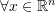: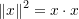#### Euclidian Dot Product in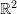and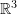In two and three dimensional vector spaces, the dot product is related to the geometric angle between two vectors as follows,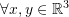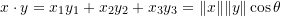where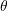is the geometric angle between the vectors x and y.

#### Orthogonal Vectors

Two vectors are called “orthogonal” if their inner product is equal to zero. For Euclidean vector spaces, the term: “perpendicular” is used interchangeably with “orthogonal”.

##### Exercises:
• Show that